StatLect

# Geometric distribution

The geometric distribution is the probability distribution of the number of failures we get by repeating a Bernoulli experiment until we obtain the first success.## Intuition

Consider a Bernoulli experiment, that is, a random experiment having two possible outcomes: either success or failure.

We repeat the experiment until we get the first success, and then we count the numberof failures that we faced prior to recording the success.

Since the experiments are random,is a random variable.

If the repetitions of the experiment are independent of each other, then the distribution ofis called geometric distribution.

Example If we toss a coin until we obtain head, the number of tails before the first head has a geometric distribution.

At the end of this lecture we will also study a slight variant of the geometric distribution, called shifted geometric distribution. The latter is the distribution of the total number of trials (all the failures + the first success).

In other words, ifhas a geometric distribution, thenhas a shifted geometric distribution.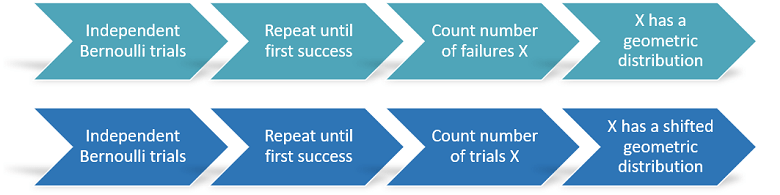## Definition

The geometric distribution is characterized as follows.

Definition Letbe a discrete random variable. Let. Let the support ofbe the set of non-negative integersWe say thathas a geometric distribution with parameterif its probability mass function isThe following is a proof thatis a legitimate probability mass function.

Proof

The probabilitiesare well-defined and non-negative for anybecause. We just need to prove that the sum ofover its support equals:where in stepwe have used the formula for geometric series.

## Relation to the Bernoulli distribution

Remember that a Bernoulli random variable is equal to:

•(success) with probability;

•(failure) with probability.

The following proposition shows how the geometric distribution is related to the Bernoulli distribution.

Proposition Letbe a sequence of independent Bernoulli random variables with parameter. Then, for any integer, the probability thatforandiswhereis the probability mass function of a geometric distribution with parameter.

Proof

Since the Bernoulli random variables are independent, we have that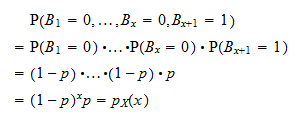## Expected value

The expected value of a geometric random variableisProof

It can be derived as follows: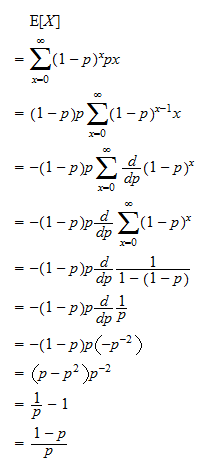## Variance

The variance of a geometric random variableisProof

Let us first derive the second moment of: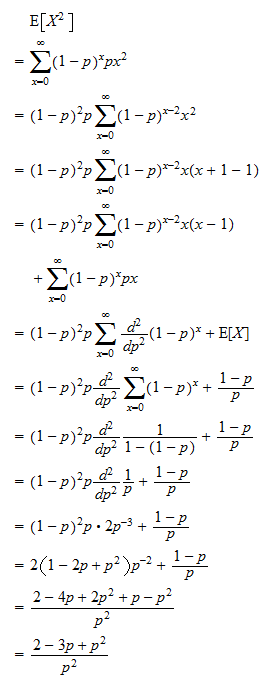Now, we can use the variance formula: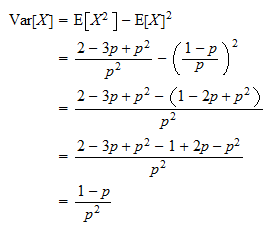## Moment generating function

The moment generating function of a geometric random variableis defined for any:Proof

This is proved as follows:where the series in stepconverges only ifthat is, only ifBy taking the natural log of both sides, the condition becomes## Characteristic function

The characteristic function of a geometric random variableis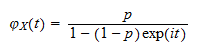Proof

The proof is similar to the proof for the mgf:## Distribution function

The distribution function of a geometric random variableisProof

For,, becausecannot be smaller than. For, we have## The shifted geometric distribution

As we have said in the introduction, the geometric distribution is the distribution of the number of failed trials before the first success.

The shifted geometric distribution is the distribution of the total number of trials (all the failures + the first success).

In other words, ifhas a geometric distribution, thenhas a shifted geometric distribution.

It is then simple to derive the properties of the shifted geometric distribution.

It expected value isIts variance isIts moment generating function is, for any:Its characteristic function is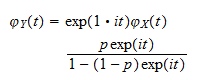Its distribution function is## Relation to the exponential distribution

The geometric distribution is considered a discrete version of the exponential distribution.

Suppose that the Bernoulli experiments are performed at equal time intervals.

Then, the geometric random variableis the time (measured in discrete units) that passes before we obtain the first success.

Contrast this with the fact that the exponential distribution is used to model the time elapsed before a given event occurs when time is continuous.

From a mathematical viewpoint, the geometric distribution enjoys the same memoryless property possessed by the exponential distribution:

• in the exponential case, the probability that the event happens during a given time interval is independent of how much time has already passed without the event happening;

• in the geometric case, the probability that the event happens at a given point in (discrete) time is not dependent on what happened before; in fact, the Bernoulli experiment performed at each point in time is independent of previous trials.

## Solved exercises

Below you can find some exercises with explained solutions.

### Exercise 1

On each day we play a lottery in which the probability of winning is.

What is the expected value of the number of days that will elapse before we win for the first time?

Solution

Each time we play the lottery, the outcome is a Bernoulli random variable (equal to 1 if we win), with parameter. Therefore, the number of days before winning is a geometric random variable with parameter. Its expected value is